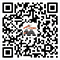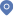Maximum bound principles and stabilized exponential time differencing schemes for conservative Allen-Cahn equations首页 - 学术活动

Lili Ju, Professor, Department of Mathematics University of South Carolina

Tao Cui, Professor

Maximum bound principles and stabilized exponential time differencing schemes for conservative Allen-Cahn equations

16:00-17:00 June 9 (Friday), N802

In comparison with the Cahn-Hilliard equation, the classic Allen-Cahn equation satisfies the maximum bound principle (MBP) but fails to conserve the total mass along the time. In this talk, we are concerned with MBPs and corresponding MBP-preserving numerical schemes for two types of modified Allen-Cahn equations that conserve the mass exactly. One is formed by introducing a nonlocal Lagrange multiplier to enforce the mass conservation, and the other is achieved through a Lagrange multiplier with both nonlocal and local effects. We propose first and second order (in time) stabilized exponential time differencing schemes for solving these two conservative Allen-Chan equations. The proposed schemes are linear and shown to be unconditionally MBP-preserving in the discrete sense. Optimal error estimates and energy stability are also obtained. We finally present various numerical experiments in two and three dimensions to validate the theoretical results and demonstrate the performance of the proposed schemes.